https://laffitto.top 乱谈府 ——詹詹碎言 2021-06-12T13:26:07.430Z https://github.com/jpmonette/feed Aspire to inspire until I expire 🎸 也扯淡，也思考，也生活 https://laffitto.top/images/avatar.png https://laffitto.top/favicon.ico All rights reserved 2021, 乱谈府 ——詹詹碎言 <![CDATA[校园霸凌]]> https://laffitto.top/post/xiao-yuan-ba-ling/ 2021-06-08T11:47:49.000Z 一些虚幻与现实的回想

]]>

]]>
<![CDATA[集成模型]]> https://laffitto.top/post/ji-cheng-mo-xing/ 2021-05-22T11:02:41.000Z 集成模型简单的总结，后续有时间再继续完善

]]>

• Bagging：这类方法的代表是随机森林
• Boosting：这类方法的代表是GBDT和XGBoost

## Bagging

Bagging主要是通过平均带来方差的减少（variance reduction）。假设有N个模型，每个模型再预测时的方差为$\sigma^2$ 。则通过N个模型一起预测时的方差是（平均）：$\frac {\sigma^2} N$

Random Forests = Bagging w. trees + random feature subsets

## Boosting

Boosting算法本质是通过学习一个个弱分类器，在之前分类器的基础上不断迭代，最终集成提升为强分类器。boosting方法大致可以分为两种：

• Adaptive boosting: 通过分类误差率来调整样本的权重，经过迭代不断更新样本权重（提高错误样本权重，降低正确样本权重）（同时适用于基分类器）。最后将多个分类器组合在一起。
• Gradient boosting : 基于残差来优化，不断更新迭代。最后将多个分类器组合在一起。

### CART回归树

GBDT使用的决策树基础是CART回归树，由于其每次迭代要拟合的是梯度值，属于连续值，所以不可以使用CART分类树。为了很好的评判拟合程序，选择使用平方误差（MSE）。

（1）选择最优切分变量$j$与切分点$s$，求解

$\min _{j, s}\left[\min _{c_{1}} \sum_{x_{i} \in R_{1}(j, s)}\left(y_{i}-c_{1}\right)^{2}+\min _{c_{2}} \sum_{x_{i} \in R_{2}(j, s)}\left(y_{i}-c_{2}\right)^{2}\right]$

（2）用选定的对$(j,s)$划分区域并决定相应的输出值：

$R_{1}(j, s)=x\left|x^{(j)} \leq s, R_{2}(j, s)=x\right| x^{(j)}>s$

$\hat{c_{m}}=\frac{1}{N} \sum_{x_{1} \in R_{m}(j, s)} y_{i}, x \in R_{m}, m=1,2$

（3）继续对两个子区域调用步骤（1）和（2），直至满足停止条件（特定迭代次数或值满足某些条件）。
（4）将输入空间划分为$M$个区域 $R_1,R_2,...R_M$ ，生成决策树：

$f(x)=\sum_{m=1}^{M} \hat{c}_{m} I\left(x \in R_{m}\right)$

### 提升树

(1) 初始化$f_0(x)=0$

(2) For $m=1,2,……M$

​ (a) 针对每个样本$(x_i,y_i)$，计算残差

$r _ { m , i } = y _ { i } - f _ { m - 1 } ( x _ { i } ) , i = 1 , 2 , \cdots , N$

​ (b) 利用 $\left\{\left(x_{i}, r_{m, i}\right)\right\}_{i=1,2, \ldots, N}$ 训练出一个决策树（回归树），得到$T ( x ; \Theta _ { m } )$

​ (c) 更新$f_{m}(x)=f_{m-1}(x)+T\left(x ; \Theta_{m}\right)$

(3) 完成以上迭代，即可得到提升树

$f_{M}(x)=\sum_{m=1}^{M} T\left(x ; \Theta_{m}\right)$

### GBDT

GBDT（Gradient Boosting Decision Tree），梯度提升树。结合上面两种思想构建而成。

GBDT是基于 Boosting的思想，串行地构造多棵决策树来进行数据的预测，对损失函数做梯度下降，每轮迭代都去拟合损失函数在当前模型下的负梯度，把待求的決策树模型当作参数，从而使得参数朝着最小化损失函数的方向更新。

GBDT算法

(1) 初始化 $f_{0}(x)=\arg \min _{c} \sum_{i=1}^{N} L\left(y_{i}, c\right)$

(2) For $m=1,2,……M$

​ (a) 针对每个样本$(x_i,y_i)$，计算残差

$r_{m, i}=-\left[\frac{\partial L\left(y_{i}, f\left(x_{i}\right)\right)}{\partial f\left(x_{i}\right)}\right]_{ \left.f(x)=f_{m-1}(x)\right)}, i=1,2, \ldots, N$

​ (b) 利用$\left\{\left(x_{i}, r_{m, i}\right)\right\}_{i=1,2, \ldots, N}$ 训练出第$m$棵回归树$T_m$，其叶节点划分的区域为$R_{m, j}, j=1,2, \ldots, J$

​ (c) 对于回归树的每一个叶节点，计算器输出值

$c_{m, j}=\arg \min _{c} \sum_{x_{i} \in R_{m, j}} L\left(y_{i}, f_{m-1}\left(x_{i}\right)+c\right), j=1,2, \ldots, J$

​ (d) 更新$f_{m}(x)=f_{m-1}(x)+\sum_{j=1}^{J} c_{m, j} I\left(x \in R_{m, j}\right)$

(3) 得到最终的梯度提升回归树

$\hat{f}(x)=f_{M}(x)=\sum_{m=1}^{M} \sum_{j=1}^{J} c_{m, j} I\left(x \in R_{m, j}\right)$

### XGBoost

XGBoost（eXtreme Gradient Boosting） 极端梯度提升，既可运用于分类，也可运用于回归。

XGBoost是对GBDT的进一步改进。传统GBDT在优化时只用到一阶导数信息， Xgboost则对损失函数进行了二阶泰勒展开，同时用到了一阶和二阶导数。
XGBOOST在损失函数里加入了正则项，用于控制模型的复杂度。正则项里包含了树的叶子节点个数、每个叶子节点上输出的 score的L2模的平方和。从 Bias-variance tradeoff角度来讲，正则项降低了模型的 variance,使学习出来的模型更加简单，防止过拟合，这也是 Xgboost优于传统GBDT的一个特性。

1. 如何构造目标函数

$\hat{y}_{i}=\phi\left(X_{i}\right)=\sum_{k=1}^{K} f_{k}\left(X_{i}\right), f_{k} \in \mathcal{F}$

$\mathcal{L}(\phi)=\sum_{i} l\left(\hat{y}_{i}, y_{i}\right)+\sum_{k} \Omega\left(f_{k}\right)$

$\hat{k}_{i}^{(t)}=\hat{k}_{i}(t-1)+f_{k}\left(x_{i}\right)$

\begin{aligned} \mathcal{L}(\phi) &=\sum_{i}^{n} l\left(y_{i}, \hat{y}_{i}^{(k)}\right)+\sum_{k=1}^{K} \Omega\left(f_{k}\right) \\ &=\sum_{i}^{n} l\left(y_{i}, \hat{y}_{i}^{(k-1)}+f_{k}\left(X_{i}\right)\right)+\sum_{k=1}^{K-1} \Omega\left(f_{K}\right)+\Omega\left(f_{K}\right)\end{aligned}

\begin{aligned} \mathcal{L}^{(K)} &=\sum_{i}^{n} l\left(y_{i}, \hat{y}_{i}^{(k-1)}+f_{k}\left(X_{i}\right)\right)+\Omega\left(f_{K}\right)\end{aligned}

1. 目标函数的近似

$f ( x + \Delta x ) \approx f ( x ) + f ^ { \prime } ( x ) \Delta x + \frac { 1 } { 2 } f ^ { \prime \prime } ( x ) \Delta x ^ { 2 }$

$f_k(X_i)$看作$\Delta x$（为何可以这样？因为每棵树迭代相加提升的数值很小，可以这样去理解）。则第K棵树目标函数转化为：

$\mathcal{L}^{(K)} \simeq \sum_{i=1}^{n}\left[l\left(y_{i}, \hat{y}_{i}^{(K-1)}\right)+g_{i} f_{K}\left(X_{i}\right)+\frac{1}{2} h_{i} f_{K}^{2}\left(X_{i}\right)\right]+\Omega\left(f_{K}\right)$

1. 将树的结构引入目标函数

$\Omega\left(f_{K}\right)=\gamma T+\frac{1}{2} \lambda \sum_{j=1}^{T} w_{j}^{2}$

\begin{aligned} \mathcal{L}^{(K)} & \simeq \sum_{i=1}^{n}\left[g_{i} f_{K}\left(X_{i}\right)+\frac{1}{2} h_{i} f_{K}^{2}\left(X_{i}\right)\right]+\Omega\left(f_{K}\right) \\ &=\sum_{i=1}^{n}\left[g_{i} w_{q\left(x_{i}\right)}+\frac{1}{2} h_{i} w_{q\left(x_{i}\right)}^{2}\right]+\gamma T+\lambda \frac{1}{2} \sum_{j=1}^{T} w_{j}^{2} \\ &=\sum_{j=1}^{T}\left[\left(\sum_{i \in I_{j}} g_{i}\right) w_{j}+\frac{1}{2}\left(\sum_{i \in I_{j}} h_{i}+\lambda\right) w_{j}^{2}\right]+\gamma T \end{aligned}

Tips:第二至三步：将所有节点1到n的循环改写成单独叶节点$I_J$1到T的循环（优点：去除下标，包含二次项）

$G_{j}=\sum_{i \in I_{j}} g_{i}, H_{j}=\sum_{i \in I_{j} }g_{i}$，代入上式：

$\mathcal{L}^{(K)}=\sum_{j=1}^{T}\left[G_{j} w_{j}+\frac{1}{2}\left(H_{j}+\lambda\right) w_{j}^{2}\right]+\gamma T$

$w _ { j } ^ { * } = - \frac { G _ { j } } { H _ { j } + \lambda }$

$L ^ { ( K ) } = - \frac { 1 } { 2 } \sum _ { j = 1 } ^ { T } \frac { G _ { j } ^ { 2 } } { H _ { j } + \lambda } + \gamma T$

1. 优化方法（使用贪心算法）

$G a i n=\frac{1}{2}\left[\frac{G_{L}^{2}}{H_{L}+\lambda}+\frac{G_{R}^{2}}{H_{R}+\lambda}-\frac{\left(G_{L}+G_{R}\right)^{2}}{H_{L}+H_{R}+\lambda}\right]-\gamma$

$\mathcal{L}_{\text {split }}=\frac{1}{2}\left[\frac{\left(\sum_{i \in I_{L}} g_{i}\right)^{2}}{\sum_{i \in I_{L}} h_{i}+\lambda}+\frac{\left(\sum_{i \in I_{R}} g_{i}\right)^{2}}{\sum_{i \in I_{R}} h_{i}+\lambda}-\frac{\left(\sum_{i \in I} g_{i}\right)^{2}}{\sum_{i \in I} h_{i}+\lambda}\right]-\gamma$

XGBoost同样有很多优化，例如Shrinkage，列抽样，稀疏值处理等等，也不细展开了，具体详见论文。

## 参考

GBDT算法原理以及实例理解

XGBoost论文解读

AdaBoost、GBDT、RF、XGboost、LightGBM的对比分析

XGBoost原始论文：XGBoost: A Scalable Tree Boosting System

]]>
<![CDATA[信息时代的内容与旁观者]]> https://laffitto.top/post/xin-xi-shi-dai-de-nei-rong-yu-pang-guan-zhe/ 2021-05-09T08:08:14.000Z 内容变化，立场也发生了变化。

]]>

「当局者清，旁观者迷」

]]>
<![CDATA[汕头之旅]]> https://laffitto.top/post/shan-tou/ 2021-04-27T11:51:55.000Z 旅行小记录

]]>]]>
<![CDATA[《巴拉巴西成功定律》读书笔记]]> https://laffitto.top/post/lesslessba-la-ba-xi-cheng-gong-ding-lu-greatergreater-du-shu-bi-ji/ 2021-04-11T13:11:22.000Z 一本用科学原理来阐述成功定义的书。

]]>

《巴拉巴西成功定律》是艾伯特-拉斯洛 · 巴拉巴西作品，作为一个科学家，他发布过很多的学术论文，取得了很大的成功。他研究复杂网络，用科学揭示了以下五条成功定律：

The First Law: Performance drives success, but when performance can't be measured, networks drive success.

The Second Law: Performance is bounded, but success is unbounded.

The Third Law: Previous success × fitness = future success.

The Fourth Law: While team success requires diversity and balance, a single individual will receive credit for the group's achievements.

The Fifth Law: With persistence success can come at any time.

## 能力表现驱动成功，但当能力表现无法被衡量时，社会网络驱动成功。

《喷泉》表明：在品质和能力表现出现内在缺失的领域里，若想理解成功，会有多么困难。

## 能力表现是有界的，但成功是无界的。

“能力表现是有界的”这句话听起来可能有些抽象，但在我所从事的量化研究领域是有清楚、准确的含义的。它意味着，我们的能力表现遵循着一个像钟形曲线的分布。

## 成功可以发生在任何时间和年龄，只要你在一个好想法上坚持不懈。

S=Qr：一个产品的成功，一个创新的影响力，等于个人的Q因子和想法的价值r的乘积。S=Qr：The success of a product or a deal, or the impact of a discovery, willbe the product of a creator's Q-factor and the value of idea r.

## 最重要的是初始的成功

]]>
<![CDATA[排序算法整理]]> https://laffitto.top/post/pai-xu-suan-fa-zheng-li/ 2021-03-27T08:59:48.000Z Algorithms 4th的排序算法整理

]]>
Algorithms 4th的排序算法整理

[TOC]

## 排序算法总体比较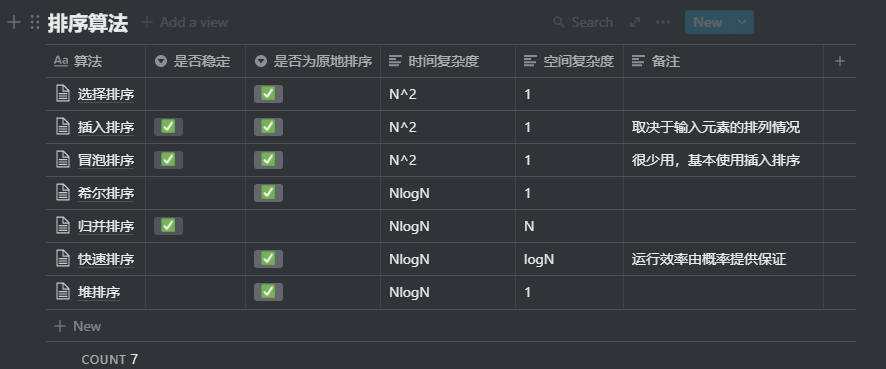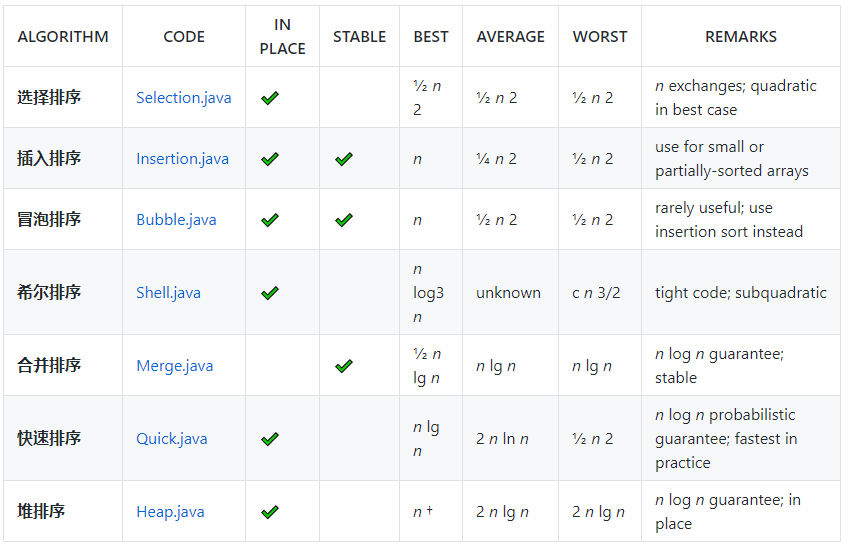### 稳定性

• 稳定的排序算法：插入排序、归并排序
• 不稳定的排序算法：选择排序、希尔排序、快速排序和堆排序

## 插入排序(Insertion sort)

（1）设待排序数组a[n]，默认a是一个有序序列
（2）循环n-1次，每次将为排序序列插入到前面的已排序序列当中，将已排序序列区间长度加1，未排序区间长度减一
（3）重复（2）直到未排序区间长度为0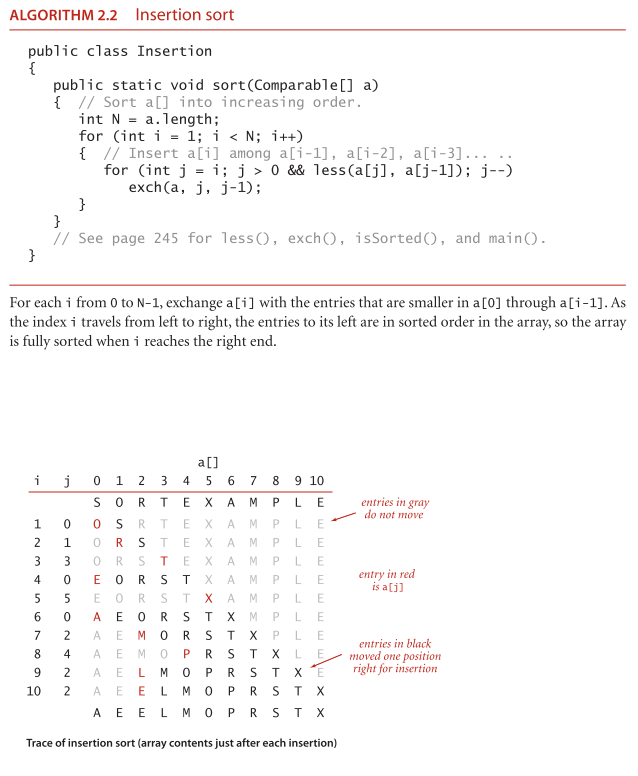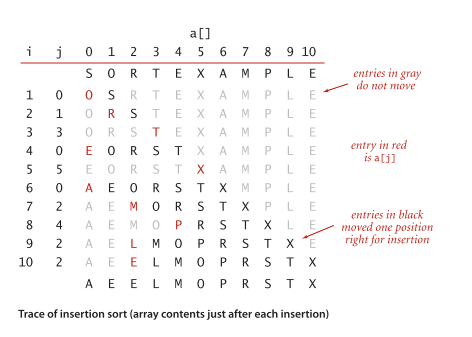java代码：

    // 插入排序
public void insertSort(int[] nums) {
if (nums == null || nums.length == 0)
return;

// 假设第一个数位置是正确的
for (int i = 1; i < nums.length; i++) {
int j = i;

int target = nums[j];

// 后移
while (j > 0 && nums[j - 1] > target) {
nums[j] = nums[j - 1];
j--;
}

// 插入
nums[j] = target;
}
}


python代码：

def InsertSort(ls):
n=len(ls)
if n<=1:
return ls
for i in range(1,n):
j=i
target=ls[i]            #每次循环的一个待插入的数
while j>0 and target<ls[j-1]:       #比较、后移，给target腾位置
ls[j]=ls[j-1]
j=j-1
ls[j]=target            #把target插到空位
return ls


## 选择排序(Selection sort)

（1）设待排序的记录存放在数组r[n]中，第一趟从r开始，通过n-1次比较，从n个记录中选取最小的记录，记为r[k]，交换r和r[k]
（2）第二趟从r开始，通过n-2次比较，从n-1个记录中选出关键字最小的记录，记为r[k]，交换r和r[k]
（3）以此类推，第i趟从r[i]开始，通过n-i次比较，从n-i+1个记录中选取最小关键字记录，记为r[k]，交换 r[i]和r[k]
（4）经过n-1趟，排序完成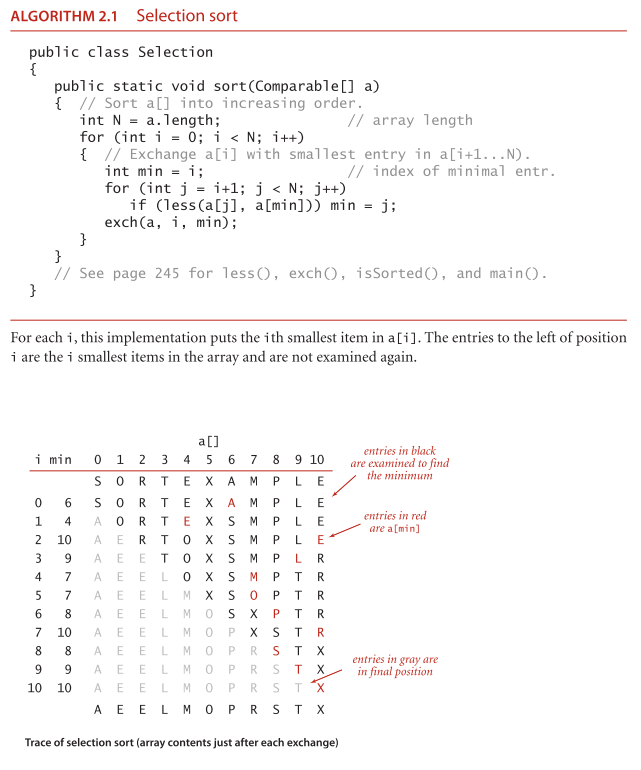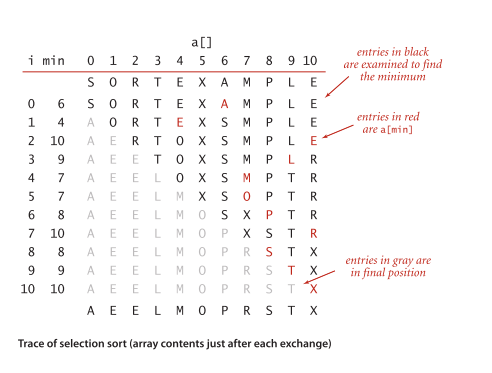java代码：

// 选择排序
public void selectSort(int[] nums) {
if (nums == null || nums.length == 0)
return;

int minIndex;

// 只需要循环 n-1 次
for (int i = 0; i < nums.length - 1; i++) {
minIndex = i;

for (int j = i + 1; j < nums.length; j++) {
if (nums[j] < nums[minIndex]) {
minIndex = j;
}
}
//交换两个值
if (minIndex != i) {
swap(nums, i, minIndex);
}
}
}


python代码：

def SelectSort(ls):
n=len(ls)
if n<=1:
return ls
for i in range(0,n-1):
minIndex=i

for j in range(i+1,n):          #比较一遍，记录索引不交换
if ls[j]<ls[minIndex]:
minIndex=j

if minIndex!=i:                     #按索引交换
(ls[minIndex],ls[i])=(ls[i],ls[minIndex])
return ls


• 运行时间和输入无关：一个已经有序的数组或是主键全部相等的数组和一个元素随机排列的数组所用的排序时间一样长；
• 数据移动是最少的：每次交换都会改变两个数组元素的值，因此选择排序用了 N 次交换——交换次数和数组的大小是线性关系。其他算法大多都是线性对数或是平方级别。

## 冒泡排序(Bubble sort)

（1） 比较两个相邻元素a[i]和a[i+1]，如果a[i]>a[i+1]，就交换这两个数的位置；
（2）重复执行第一步，直到比较到最后一对的时候（例：首次是a和a，此时，a的值为该数组的最大值，这个值属于有序数列）；
（3）对所有元素（除了有序数列里的元素），重复执行第一步和第二步，每执行完一次，都会找到当前比较的数里最大的那个（有序数列就会增加一个）；
（4）随着参与比较的元素越来越少，最终没有任何一对元素需要比较的时候，排序完成。

java代码：

    // 冒泡排序
public void bubbleSort(int[] nums) {
if (nums == null || nums.length == 0)
return;

// 只需要循环 n-1 次
for (int i = 0; i < nums.length - 1; i++) {
for (int j = 0; j < nums.length - i - 1; j++) {
if (nums[j] > nums[j + 1]) {
swap(nums, j, j + 1);
}
}
}
}


python代码

def BubbleSort(ls):
n=len(ls)
if n<=1:
return ls
for i in range (0,n):
for j in range(0,n-i-1):
if ls[j]>ls[j+1]:
(ls[j],ls[j+1])=(ls[j+1],ls[j])
return ls


## 希尔排序(Shell sort)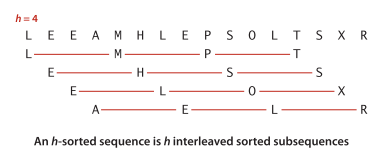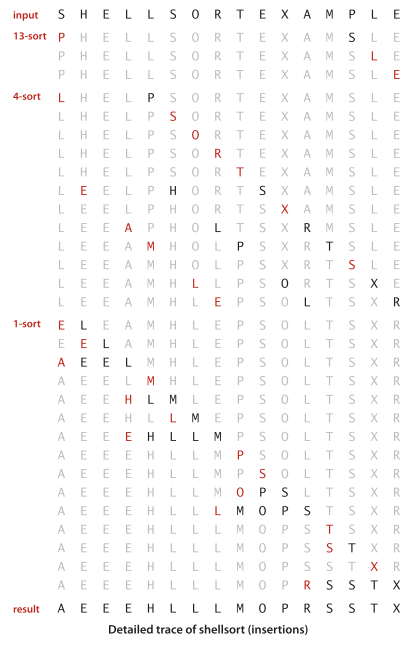public static void sort(Comparable[] a) {
// 将 a[] 按升序排列
int N = a.length;
int h = 1;
while(h < N / 3)
h = 3 * h + 1;    // 1, 4, 13, 40, 121, 364, 1093, ...
while(h >= 1) {
// 将数组变为 h 有序
for(int i = h; i < N; i++) {
// 将 a[i] 插入到 a[i-h]，a[i-2*h]，a[i-3*h]... 之中
for(int j = i; j >= h && less(a[j], a[j -h]); j -= h)
exch(a, j, j-h);
}
h /= 3;
}
}


## 归并排序(Merge sort)

（1）将当前序列一分为二，求出分裂点mid=(l+r)/2
（2）对子序列r[l]-r[mid]递归，进行归并排序，结果放在t[l,mid]中
（3）对子序列r[mid+1,r]递归，进行归并排序，结果放在t[mid+1,r]
（4）将两个有序的子序列t[l,mid]，t[mid+1,r]归并为一个有序的序列放入r[l,r]中

## merge思想图解：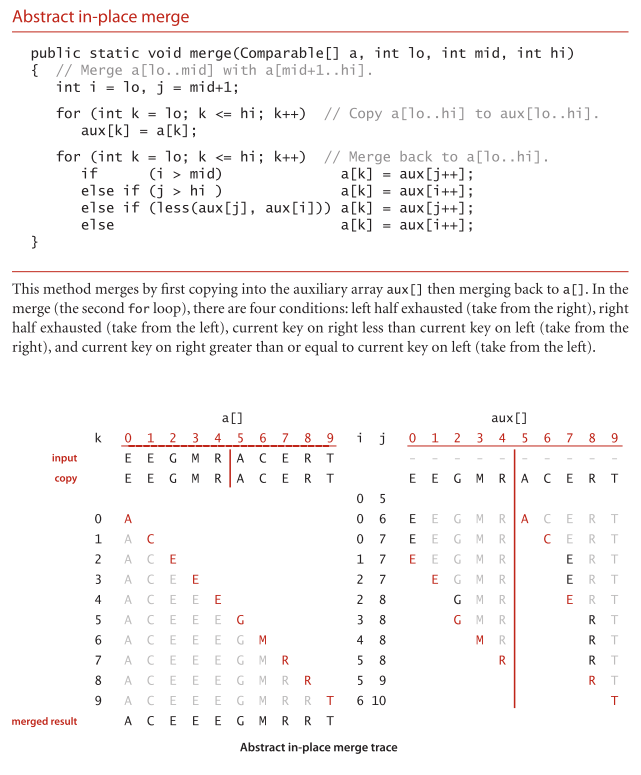## 自顶而下归并排序（递归）

java代码：

    // 归并排序
public void mergeSort(int[] arr, int start, int end) {
if (start >= end)
return;

int mid = (start + end) / 2;

// 递归排序左边
mergeSort(arr, start, mid);
// 递归排序右边
mergeSort(arr, mid + 1, end);

// 合并
merge(arr, start, mid, end);
}

// 合并两个有序数组
public void merge(int[] arr, int start, int mid, int end) {
int[] temp = new int[end - start + 1]; // 中间数组

int i = start;
int j = mid + 1;
int k = 0;
while (i <= mid && j <= end) {
if (arr[i] <= arr[j]) {
temp[k++] = arr[i++];
} else {
temp[k++] = arr[j++];
}
}

while (i <= mid) {
temp[k++] = arr[i++];
}

while (j <= end) {
temp[k++] = arr[j++];
}

for (p = start; p <= end; p++) {
arr[p] = temp[p];
}

/*     for (int p = 0; p < temp.length; p++) {
arr[start + p] = temp[p];
}
*/
}


//归并排序
public void sort(int[] arr,int start,int end){
if(start >= end)return;

int mid = start + (end-start)/2;
sort(arr,start,mid);
sort(arr,mid+1,end);
merge(arr,start,mid,end);
}

//合并
public void merge(int[] arr, int start, int mid, int end) {
int i=start,j=mid+1,k=0;
int[] temp = new int[end-start+1];

for(int k=start;k<=end;k++){
if(i>mid)temp[k]=arr[j++];
else if(j>end)temp[k]=arr[i++];
else if(arr[i]>arr[j])temp[k] = arr[j++];
else temp[k] = arr[i++];
}
}


python代码：

def MergeSort(ls):
#合并左右子序列函数
def merge(arr,left,mid,right):
temp=[]     #中间数组
i=left          #左段子序列起始
j=mid+1   #右段子序列起始
while i<=mid and j<=right:
if arr[i]<=arr[j]:
temp.append(arr[i])
i+=1
else:
temp.append(arr[j])
j+=1
while i<=mid:
temp.append(arr[i])
i+=1
while j<=right:
temp.append(arr[j])
j+=1
for i in range(left,right+1):    #  !注意这里，不能直接arr=temp,他俩大小都不一定一样
arr[i]=temp[i-left]

#递归调用归并排序
def mSort(arr,left,right):
if left>=right:
return
mid=(left+right)//2
mSort(arr,left,mid)
mSort(arr,mid+1,right)
merge(arr,left,mid,right)

n=len(ls)
if n<=1:
return ls
mSort(ls,0,n-1)
return ls


## 自底向上的归并排序（非递归）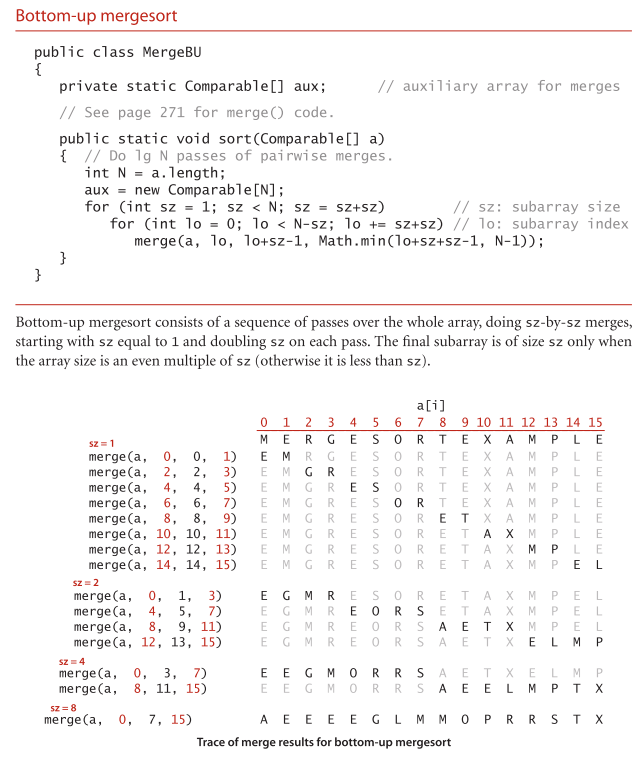## 快速排序(Quick sort)

• 原地排序（只需要一个很小的辅助栈）；
• 将长度为 N 的数组排序所需的时间和 NlgN 成正比。

（1）选择待排序记录中第一个记录作为基准，将基准暂存在r的位置上，假设两个指针low和high，初始分别指向表的下界和上界
（2）从表的最右侧位置向左寻找第一个比基准数小的位置，从表的最左侧寻找第一个比基准数大的位置，交换两个元素
（3）重复（2）当low==high时，将基准数放到low位置处的元素判断大于还是小于后，与基准数交换
（4）此时以基准数的位置为分界点，将序列分为两个子序列，分别进行（1），（2），（3）操作，直到序列的长度为1为止

java代码：

    // 快速排序
public void quickSort(int[] nums, int start, int end) {
if (start >= end)
return;

int partitionIdx = partition(nums, start, end);

quickSort(nums, start, partitionIdx - 1);
quickSort(nums, partitionIdx + 1, end);
}

// partition
public int partition(int[] nums, int start, int end) {
if (start == end) {
return start;
}

int pivot = nums[start];

while (start < end) {
// 从右往左找到第一个小于 pivot 的元素
while (start < end && nums[end] >= pivot) {
end--;
}
// 把小的移动到左边
nums[start] = nums[end];

// 从左往右找到第一个大于 pivot 的元素
while (start < end && nums[start] <= pivot) {
start++;
}
// 把大的移动到右边
nums[end] = nums[start];
}

// 最后把pivot赋值到中间
nums[start] = pivot;

return start;
}


python代码：

def QuickSort(ls):
def partition(arr,left,right):
key=left         #划分参考数索引,默认为第一个数，可优化
while left<right:
while left<right and arr[right]>=arr[key]:
right-=1
while left<right and arr[left]<=arr[key]:
left+=1
(arr[left],arr[right])=(arr[right],arr[left])
(arr[left],arr[key])=(arr[key],arr[left])
return left

def quicksort(arr,left,right):   #递归调用
if left>=right:
return
mid=partition(arr,left,right)
quicksort(arr,left,mid-1)
quicksort(arr,mid+1,right)
#主函数
n=len(ls)
if n<=1:
return ls
quicksort(ls,0,n-1)
return ls


## 堆排序(Heap)（优先队列 Priority queues）

（1）按照堆的定义将待排序序列a[n] 调整为大根堆，这个过程称为初建堆，交换a和a[n] ，则a[n]为关键字的最大记录
（2）将a[n-1]重新调整为堆，交换ra和a[n-1]，此时a[n-1]为关键字最大记录
（3）循环n-1次，直到交换a和a为止

python构建堆代码：

def  HeapSort(ls):
def heapadjust(arr,start,end):  #将以start为根节点的堆调整为大顶堆
temp=arr[start]
son=2*start+1
while son<=end:
if son<end and arr[son]<arr[son+1]:  #找出左右孩子节点较大的
son+=1
if temp>=arr[son]:       #判断是否为大顶堆
break
arr[start]=arr[son]     #子节点上移
start=son                     #继续向下比较
son=2*son+1
arr[start]=temp             #将原堆顶插入正确位置
#######
n=len(ls)
if n<=1:
return ls
#建立大顶堆
root=n//2-1    #最后一个非叶节点（完全二叉树中）
while(root>=0):
heapadjust(ls,root,n-1)
root-=1
#掐掉堆顶后调整堆
i=n-1
while(i>=0):
(ls,ls[i])=(ls[i],ls)  #将大顶堆堆顶数放到最后
heapadjust(ls,0,i-1)    #调整剩余数组成的堆
i-=1
return ls


]]>
<![CDATA[买了点啥数字服务？]]> https://laffitto.top/post/mai-liao-dian-sha-shu-zi-fu-wu/ 2021-03-14T01:35:26.000Z 对自己购入的数字服务的一次盘点。

]]>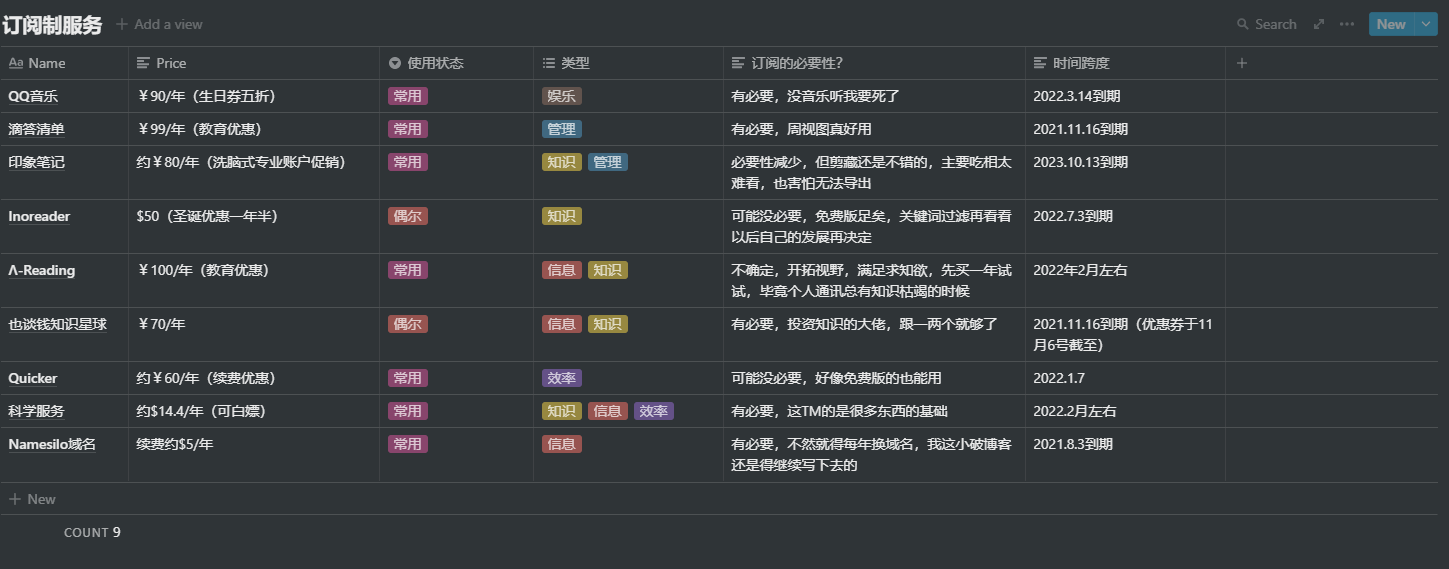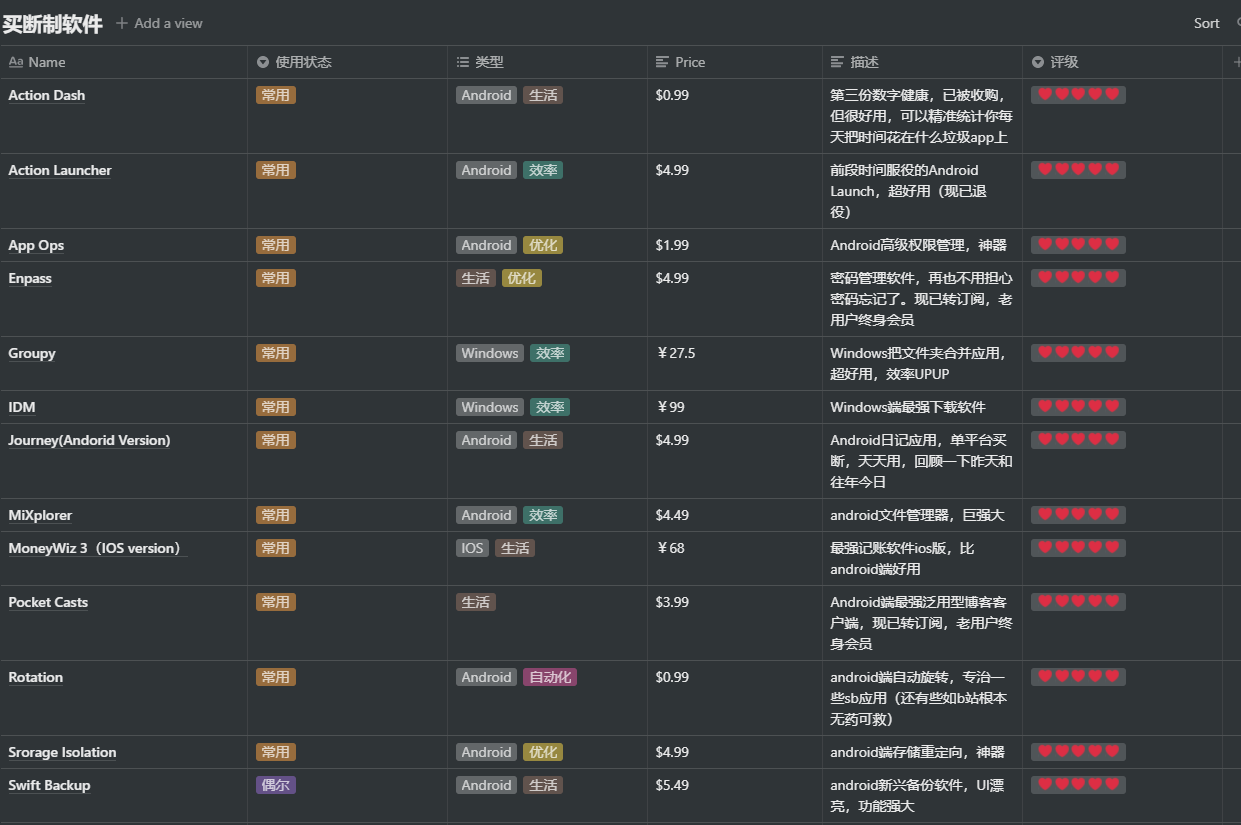All in one软件，笔记，日程，项目等等都可做，依赖于云端，目前通过教育邮箱白嫖中。非常方便，很多统计类表格都往上面搬，依赖较自由的网络环境，这既是优点也是缺点。]]>
<![CDATA[恶之华——成长的让步与妥协]]> https://laffitto.top/post/e-zhi-hua-cheng-chang-de-rang-bu-yu-tuo-xie/ 2021-03-02T12:03:08.000Z 漫画恶之华读后感想~

]]>

## 什么是恶之花？

“由于他以前做了什么什么，那么这件恶劣的事情也可能是他做的。”

“这件事你是被他带着干的吧，他是不是逼你了？我相信你自己不会做出这种事。”

“经过调查，这起事件完全是由于某茶与父母不和才酿成的悲剧，且某茶曾被抓进过派出所。”

## 妥协 or 救赎？

（漫画阅读顺序从右到左，从上到下）## 最后的结局]]>
<![CDATA[2020投资复盘]]> https://laffitto.top/post/2020-tou-zi-fu-pan/ 2021-02-13T12:33:37.000Z 简单整理一下为之后打好基础

]]>

1. 蚂蚁财富（支付宝）

1. 且慢

1. 华宝智投

## 关于今年的计划

1. 孟岩大佬：且慢创始人，后离开且慢，创办有知有行app。跟踪方式：公众号，微博，有知有行
2. E大：且慢长赢计划的主持人。跟踪方式：微博，公众号
3. 也谈钱：科普者？带我入门的大佬。跟踪方式：公众号，知识星球

]]>
<![CDATA[小爱情]]> https://laffitto.top/post/xiao-ai-qing/ 2021-02-01T01:38:24.000Z 如同做梦的瞬间

]]>

2021年1月29号，和心怡的女生一起出来吃饭。

]]>